# Linear equationsPage 1

#### WATCH ALL SLIDES

Slide 1## Linear Equations

Linear equations are functions which have two variables. They have an independent and dependent variable.

Slide 2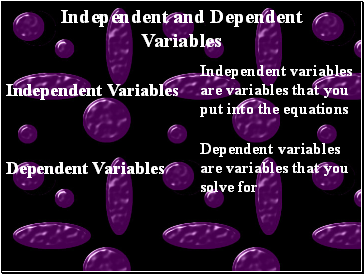## Independent and Dependent Variables

Independent Variables

Independent variables are variables that you put into the equations

Dependent Variables

Dependent variables are variables that you solve for

Slide 3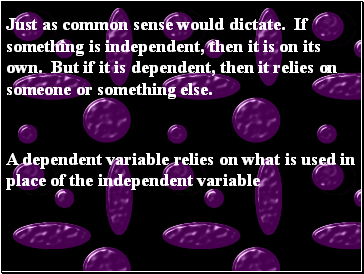Just as common sense would dictate. If something is independent, then it is on its own. But if it is dependent, then it relies on someone or something else.

A dependent variable relies on what is used in place of the independent variable

Slide 4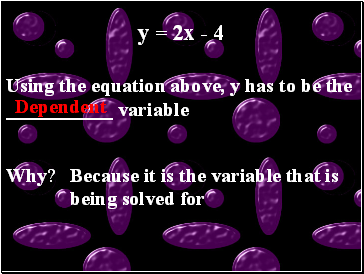y = 2x - 4

Using the equation above, y has to be the variable

Why?

Because it is the variable that is being solved for

Dependent

Slide 5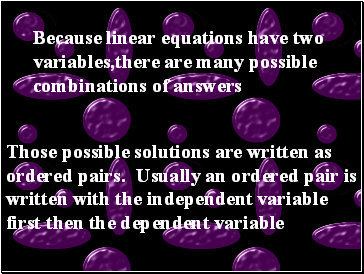Because linear equations have two variables,there are many possible combinations of answers

Those possible solutions are written as ordered pairs. Usually an ordered pair is written with the independent variable first then the dependent variable

Slide 6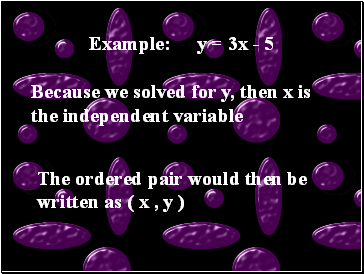Example: y = 3x - 5

Because we solved for y, then x is the independent variable

The ordered pair would then be written as ( x , y )

Slide 7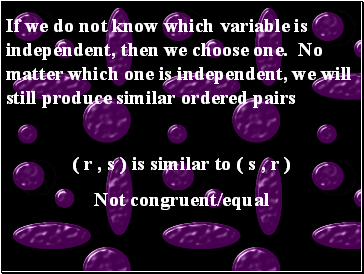If we do not know which variable is independent, then we choose one. No matter which one is independent, we will still produce similar ordered pairs

( r , s ) is similar to ( s , r )

Not congruent/equal

Slide 8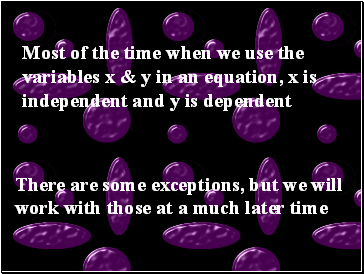Most of the time when we use the variables x & y in an equation, x is independent and y is dependent

There are some exceptions, but we will work with those at a much later time

Slide 9As said before, there are many possible ordered pairs

To find more than one solution, we use a Table of Values

Slide 10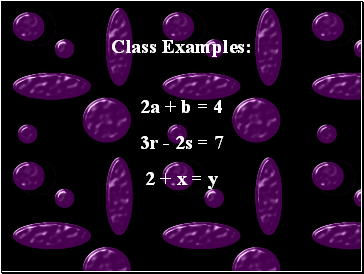Class Examples:

2a + b = 4

3r - 2s = 7

2 + x = y